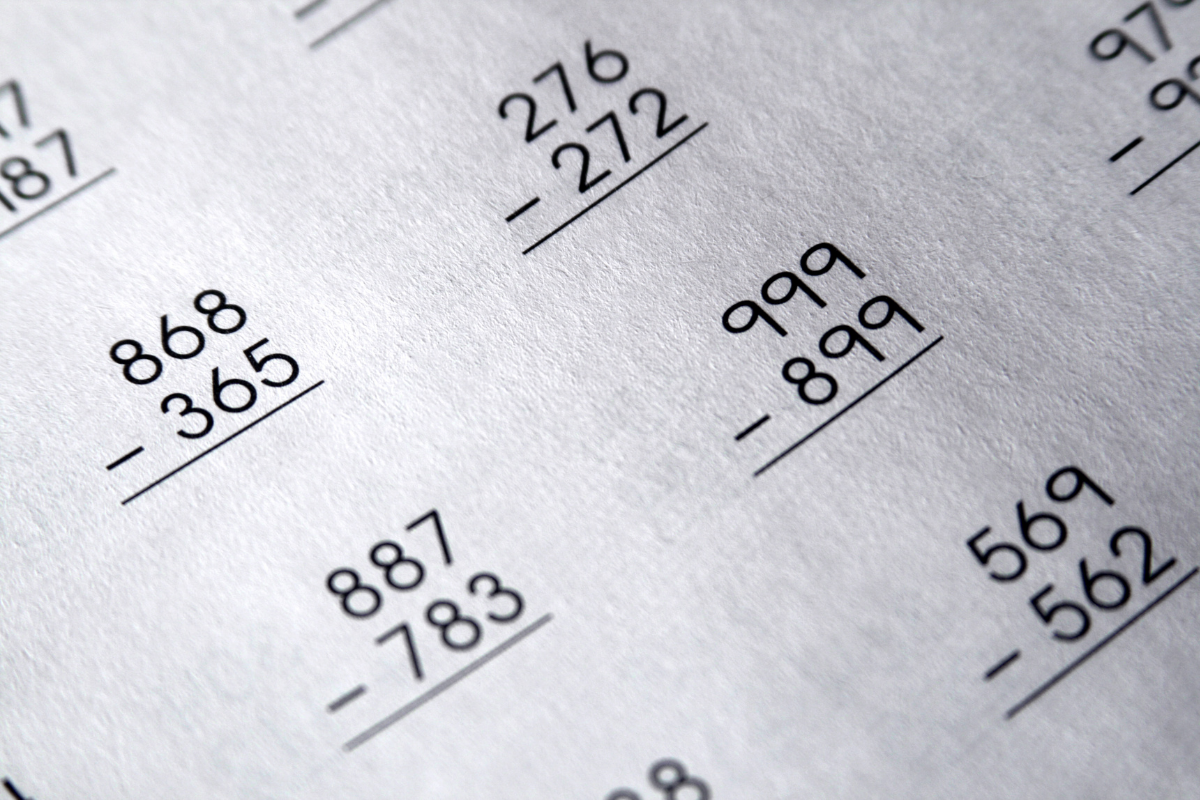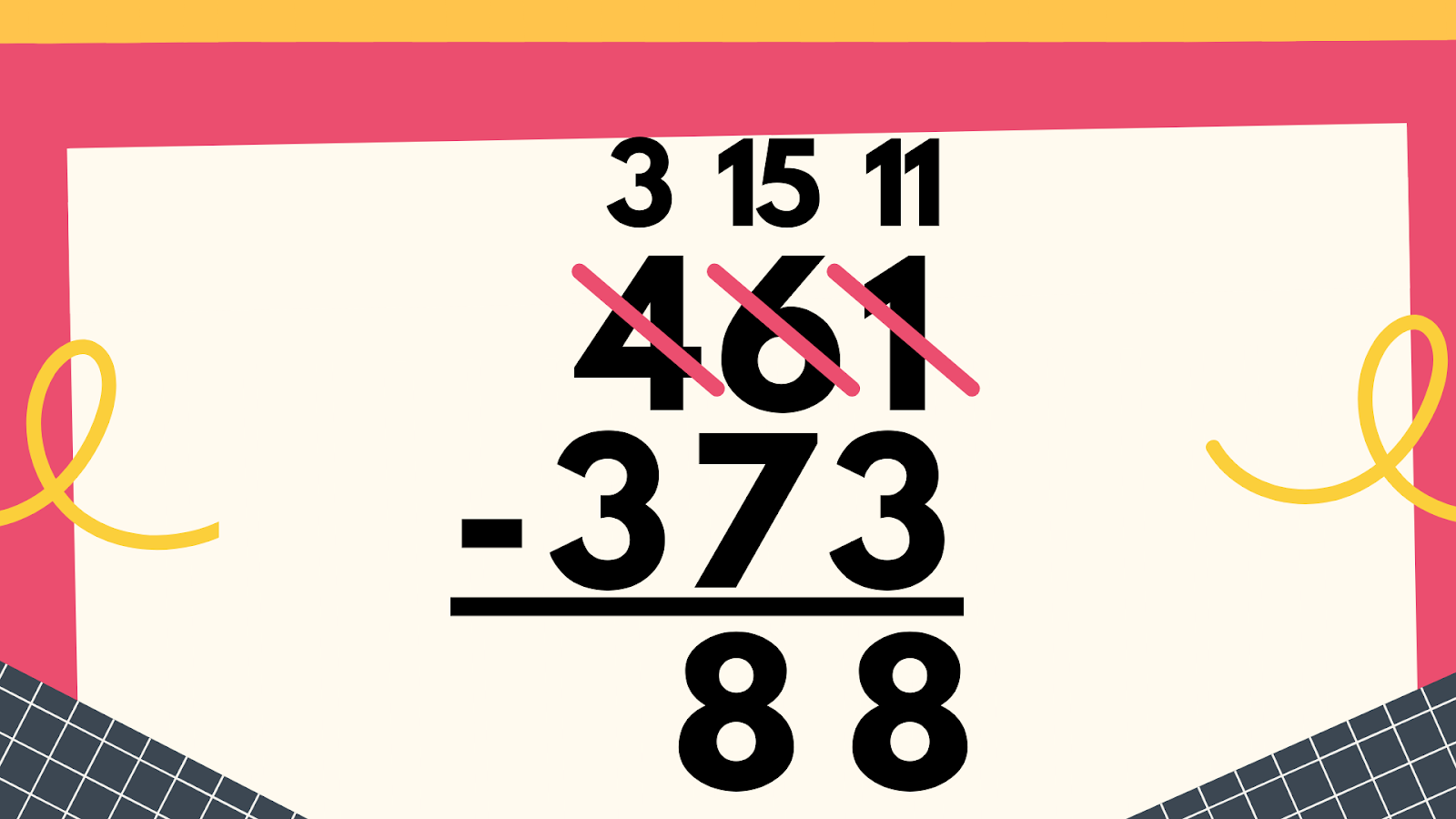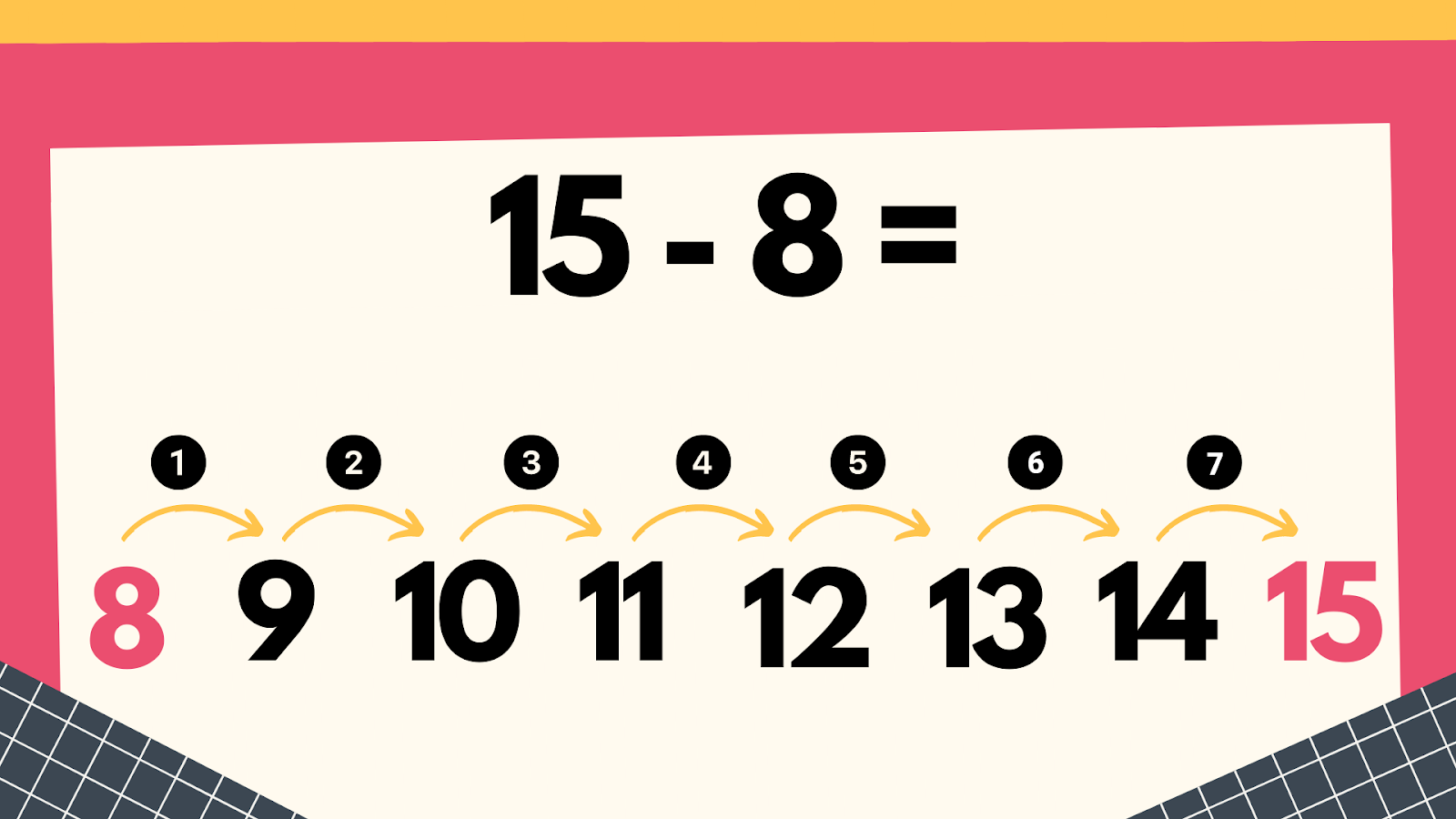Subtraction and Counting Up With Large Numbers - Exceed in Learning

# Subtraction and Counting Up With Large NumbersSubtraction is a concept that is such a struggle for some students.  As a teacher and a parent, we have always taught the standard subtraction algorithm just like what you see in the picture below.This process is easy for some students, but extremely daunting for others.  I can remember working with students on this concept in my 2nd and 3rd grade classroom.  We worked tirelessly with base ten blocks to represent problems in hopes that students could have a concrete understanding of how subtraction worked.  There were just too many steps for some of my students to remember so it was a very hard concept for them to master.

When teaching single digit subtraction, I usually always teach students the “count up” strategy.  For example, to solve 15 – 8, I encourage students to start with the number 8 and then count up until they reach 15.For some students, this proves to be much easier.  Counting forward is much easier on the brain than counting backwards.  So, with this in mind, there has to be a different way for students to master subtraction of larger numbers, right?  Let’s take a look to see if there is a way to use the count up strategy for bigger numbers.

Let’s take a look at the problem 451 – 313.  Now we certainly aren’t going to count up by ones as we did with the example before.  But, let’s see if we can break things down into hundreds, tens, and ones.

Using the number line above, I started with the smaller number and stopped at the bigger number.

·   I started with 313 and added 100 to get to 413.  I can’t “jump” another 100 because that would be 513.

·   I then jumped 30 more to land on 443.

·   Finally, I jumped 8 more numbers to land on 451.